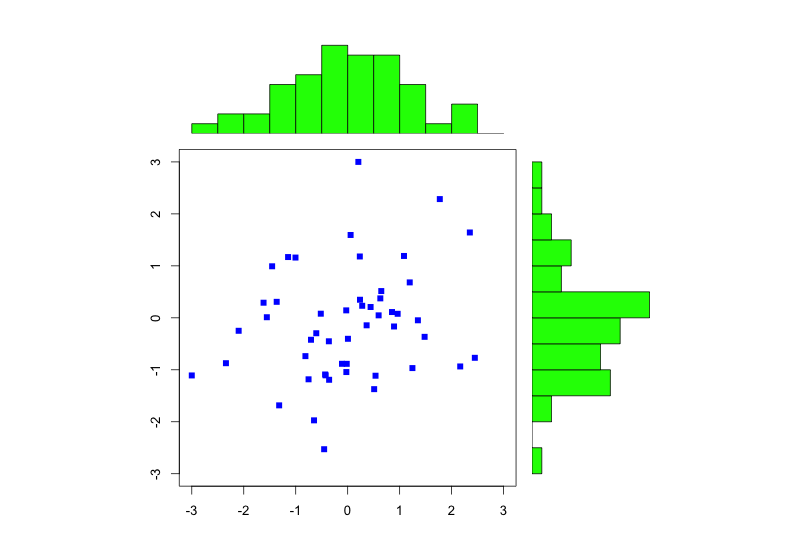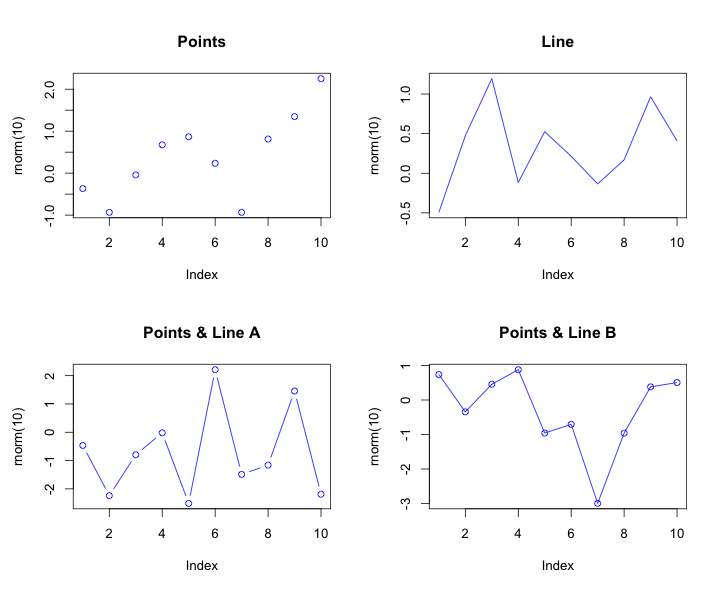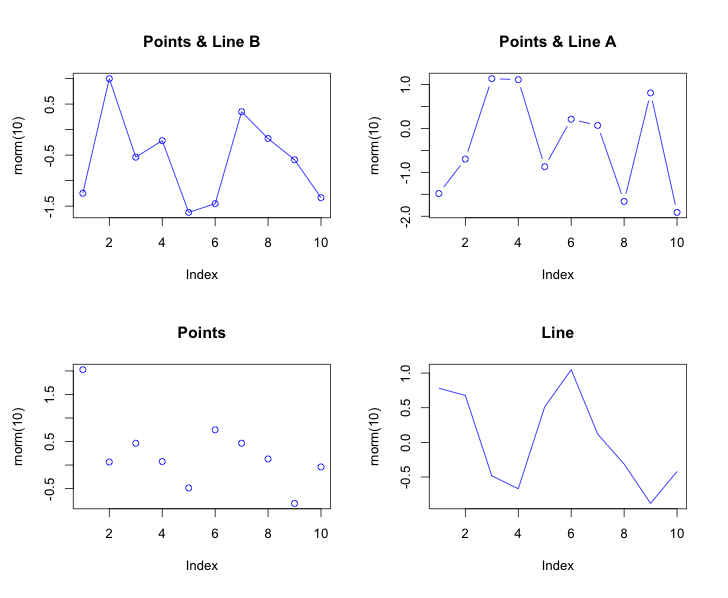# R Graphics: Multi-Graph Layouts

#### The layout() Function

The ability to manage multiple plots in one graphical device or window is a key capability to enhance data visualization and analysis.

The layout() function in base R is the most straightforward method to divide a graphical device into rows and columns.  The function requires an input matrix definition.  Column-widths and the row-heights can be defined using additional input arguments.  layout.show() can then be used to see multi-graph layouts and how the graphical device is being split.#### Using par() Arguments for Multi-Graph Layouts

The alternative method for launching multi-plots in base R is to use the functions mfrow() and mfcol(). Use just one of the functions to define a plot layout given the vector argument c(nrow, ncol) to define an nrow by ncol array on the graphical device.  The option function mfg() is then used to control plotting by array element.  Specifically, mfg() takes the argument c(i, j) , where the i and j indicate which figure in the array is to be drawn.In general, the mfrow() and mfcol() functions are much more rigid and less flexible than the layout() function.  Having said that, each approach can be useful.